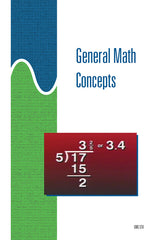Get the Study Guide for this videoFollow the video session-by-session with this handy book. Color illustrations throughout. \$15

Instructor Guide  available

General Math Concepts- Instant Video

Summary

This 40-minute video delivers a review of general mathematical concepts. It covers the four basic math functions of addition, subtraction, multiplication, and division of whole numbers, fractions, and decimals.

Training Sessions

Number Types and Addition – 7 minutes
Describes the characteristics of whole numbers, fractions, and decimal numbers. Explains what place value is and how to identify the place value of a number. Shows how whole numbers, fractions, and decimal numbers can be added. Describes one way to check the results of an addition problem. Describes how two numbers can be added using a calculator.

Subtraction – 6 minutes
Explains how whole numbers, fractions, and decimal numbers are subtracted. Describes what borrowing is and when it is necessary. Shows how fractions and decimal numbers are subtracted. Demonstrates how to check the results of a subtraction problem. Describes how two numbers can be subtracted using a calculator.

Multiplication – 7 minutes
Covers symbols used to represent multiplication operations. How to multiply whole numbers, fractions, and decimal numbers. Shows a shortcut for multiplying numbers that end in 0. How to use a calculator to multiply two numbers.

Division – 10 minutes
Coverage includes: principles of division, dividing whole numbers, dividing fractions, dividing decimal numbers, using a calculator for division.

Conversions and Special Cases – 10 minutes
Explains how to convert between fractions and decimal numbers, whole numbers and decimal numbers, and mixed numbers and improper fractions. Demonstrate how to perform operations on fractions with different denominators. Shows the order in which combined operations are performed.

Item Code: GMC-IV